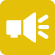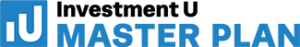## 1-Minute Survey

What Type of Investor Are You? – Take This 1-Min Survey to Find Out

Financial Literacy

# How Does Compound Interest Work?

Compound interest is the eighth wonder of the world. He who understands it, earns it; he who doesn’t, pays it.” – Albert Einstein

This quote is one many investors know well. It succinctly sums up the power of compound interest and its potential. To understand it fully, you need to ask yourself: How does compound interest work?

While many people can explain the function of compounding, they’re lost when it comes to understanding exactly how wealth begets more wealth. Do you know the formula for compound interest? Are you familiar with the “Rule of 72”? Do you know how much your investments will compound if you continue to make them on a regular schedule?

These are all questions you need to have the answers to if you want to truly understand the power of compound interest. Keep reading to get those answers.## What Is Compound Interest?

Compound interest is the interest on an invested balance that grows over time as each new calculation of that balance includes the previous interest payment. The longer the time horizon, the more opportunities for compounding. Likewise, the higher the interest rate, the faster the compounded total grows.

To understand compound interest, it’s best to pose a very simple question:

Would you rather have \$1,000,000 today or start with one penny and have the balance double every day for 30 days?

This question is popular in beginner personal finance classes. The answer is, of course, the penny doubled for 30 days. Why? Because the magic of compound interest will leave you with a balance of more than \$10.7 million on day 30 – more than 10n times the ROI of taking the million dollars upfront!

While this is an extreme example of 100% of the balance compounding, it nonetheless shows the significance of compound interest. In your retirement accounts, compound interest is a powerful tool for growing your principal investments. The sooner you start and the more you contribute, the greater that ROI becomes.

## An Example of Compounding at Work

To get a better example of compound interest, consider a more practical example. Let’s say Bailey invests a lump sum of \$50,000 at a fixed rate of 5% annually. Let’s take a look at how Bailey’s investment will grow over different time horizons if she invests another \$100 every month. After…

• One year, she’ll have \$53,786
• Five years, she’ll have \$70,968
• 10 years, she’ll have \$97,878
• 20 years, she’ll have \$176,735
• 30 years, she’ll have \$306,611.

For many investors, a six-figure total return on investment is very feasible thanks to compounding. With consistent contributions, favorable rates of return and a long enough time horizon, the opportunity for ROI is virtually limitless.

Want to see compound interest at work for your own investment? Check out our compound interest calculator to see how your contributions compound over a specific time horizon.

## The Formula for Compound Interest

As with all mathematical concepts, compound interest has a formula: P(1 +r/n)nt. In this formula…

• P = the initial principal balance
• r = the interest rate
• n = the number of times interest is applied
• t = the number of time periods elapsed.

As you can see from the raw equation, time plays a big factor in compounding. First, the frequency an amount compounds is important for raising the principal value. Second, the total amount of time invested (time periods) establishes how many times the balance grows. These two factors together are exponential, which is where the power of compound interest comes from.

## Tips to Optimize Compound Interest

Believe it or not, there are ways to get more out of compound interest. It has to do with manipulating the variables of the above equation.

Take a dividend stock, for example. You can actually double the compounding capabilities of these securities with a dividend reinvestment plan (DRIP). By reinvesting dividends, you’re compounding your principal investment in the number of shares, which yields more dividends. At the same time, when the share price appreciates, you’re also gaining wealth.

You can also optimize compounding frequency. For example, you might choose to invest in a fund that compounds monthly instead of quarterly. This doubles the number of times interest is applied.

The better you understand how compounding works, the more you’ll understand where opportunities exist to optimize it… and the easier it’ll be to capitalize on those opportunities within your preferred investing modality.

## What Is the “Rule of 72”

The Rule of 72 is a handy little formula that’s often used to guesstimate a 100% return on investment. In other words, it estimates how long it’ll take to double your money. To figure this out, divide 72 by the growth rate of your investment (or interest rate). The result is the number of years it’ll take to double your money.

The Rule of 72 also works to calculate inflation losses. Divide 72 by the expected inflation rate, and that’s how long it’ll take for your uninvested dollars to lose half their value.

## The Best Investment Vehicles for Compounding

How does compound interest work? Now that you know the answer, it’s time to use it to your advantage. That means choosing an investment vehicle that offers the best compounding prospects. For most investors, this means any type of equity security that offers dividend reinvestment opportunities.